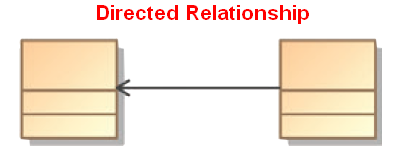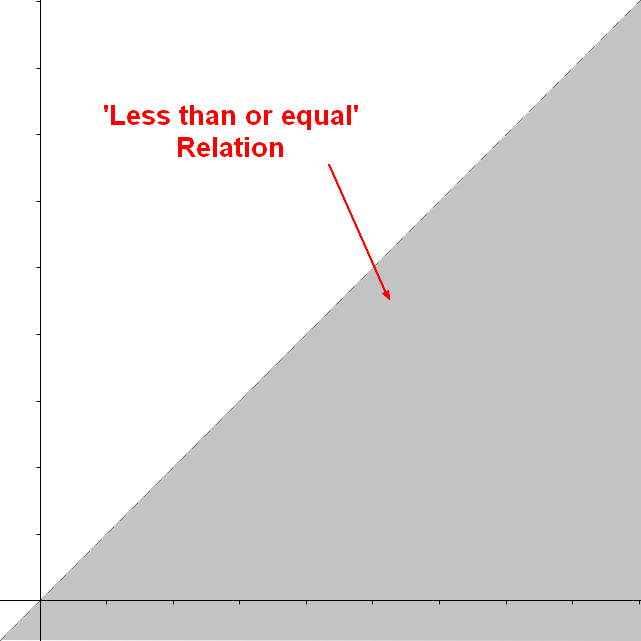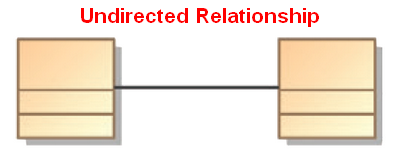# Logical Data Modeling - Antisymmetry relationship

A Antisymmetric relation is a relationship that happens when for all a and b in X:

• if a is related to b
• then b is NOT related to a
• or b=a (reflexivity is allowed)

In mathematical notation, an Antisymmetric relation between x and y follows $$y = f(x)\\ x \neq f(y) \\ x = f(y) \text{ only because } x = y$$

Or in other word, if the relation is a asymmetric

• if a is related to b
• if b is related to a
• then a = b

Every asymmetric relation is antisymmetric.

## Example

• the divisibility relation (on natural numbers): 12 is divisible by 4, but 4 is not divisible by 12
• if n and m are distinct and n is a factor of m, then m cannot be a factor of n.
• therefore the only way each of two numbers can be divisible by the other is if the two are, in fact, the same number

## Representation

### Entity Model

When there is a direction on the association, the relationship is antisymmetric.

### Set of tuple

A antisymmetric relation in the set of {1,2,3} would be the set of tuple

<1,3>
<2,1>
<3,2>
<1,1>
<2,2>
<3,3>



### Visual

• An antisymmetric and asymmetric relation between x and y (asymmetric because it is not reflexive)

Recommended PagesLogical Data Modeling - Asymmetric Relation (Uni-directional|Antisymmetric)

An asymmetric relation is a type of binary relation that requiers: antisymmetry (ie if a is related to b, b is not related to a) and irreflexivity (ie an element cannot be related to itself) irreflexivity...Logical Data Modeling - Binary Relation

A binary relation is a relationship between two elements that is implemented via a binary function. Binary relations are used in many branches of mathematics to model concepts like: order relation...Logical Data Modeling - Reflexive relationship property

reflexive is a relationship property that indicates: that the relationship relates every element to itself. in other word, that the relation set representing the relationship contains every tuple:...Logical Data Modeling - Relationship

A relationship is a model element that relates two entities . In its simple form (semantic form), it's a description that has a verb such as: has consists of uses ... entityentity setrelationship...Logical Data Modeling - Symmetric relationship (bi-directional)

A symmetric relation is a type of binary relation that happens when for all a and b in X if a is related to b, then b is related to a In mathematical notation, the relation f between x and y is...Ordinal Data - Partially Order

A Partially ordered structure is a data structure where not every pair of elements needs to be comparable (compared). A collection of people ordered by genealogical descendancy: Some pairs of...